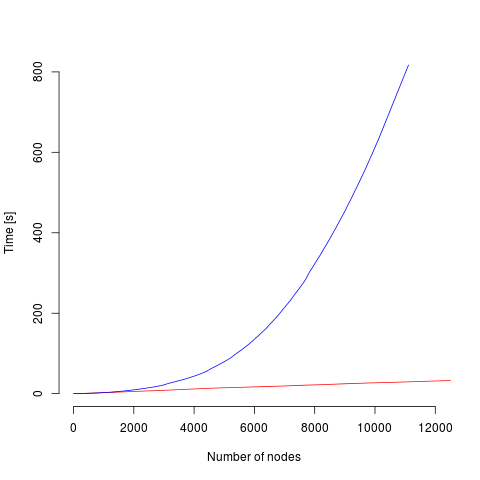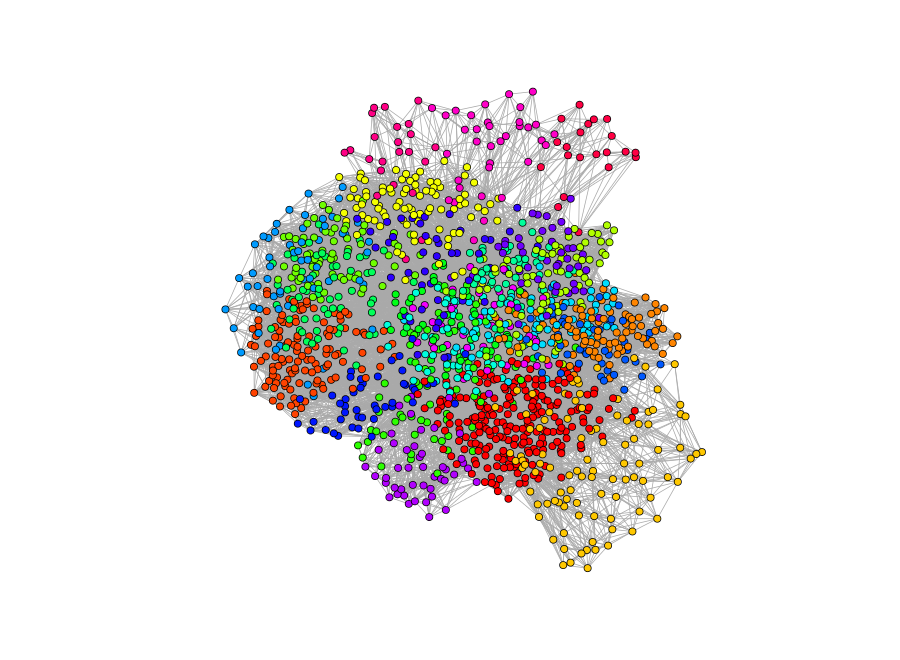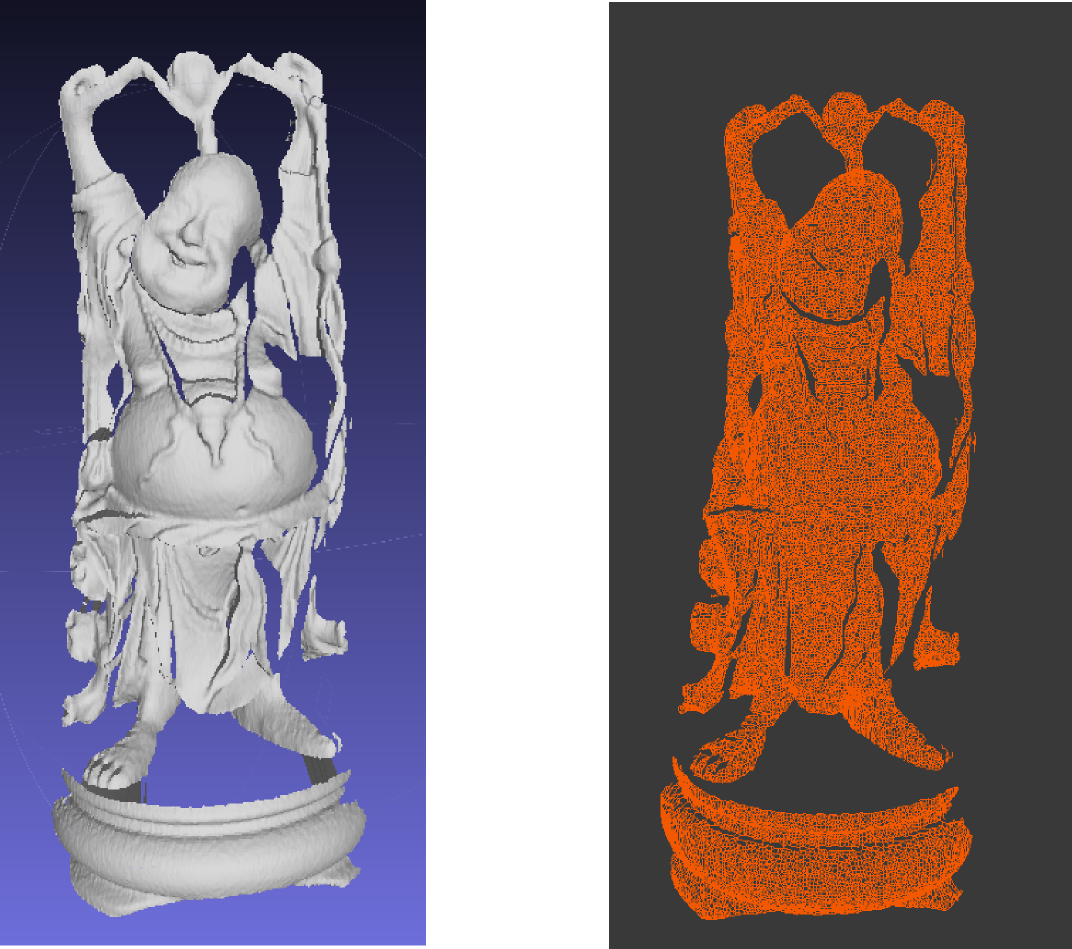## General

This package contains fast C++ implementation of online clustering algorithm Growing Neural Gas wrapped as R package using Rcpp. It produces topological graph, that you can easily convert to igraph, or you can dump your model to optimized binary file and load it later on.

This algorithms is widely used for dynamic clustering problem. Package is designed with focus on big datasets. It is already possible to cluster dataset without making its copy, with different dataset types (bagging, sequential or probability sampling). In the near future it will be possible to stream dataset from csv file.

Comparision of performance of original implementation and with improvements suggested by D. Fiser, J. Faigl, M. KulichDaniel Fiser, Jan Faigl, Miroslav Kulich optimization paper Growing neural gas efficiently

## Examples

MNIST dataset clustering (raw images)## Usage

For more detailed usage see code in demo folder, or in tests/testthat folder. You can also refer to R package documentation (pdf version gng.pdf).

### Cluster wine dataset

In this example we will construct a clustering of UCI wine dataset using offline GNG.

``````library("GrowingNeuralGas")

data(wine, package="rattle")
scaled_wine <- scale(wine[-1])

# Train in an offline manner
gng <- GNG(scaled_wine, labels=wine\$Type, max_nodes=20)

# Find closest node to vector [1,1,1]
predict(gng, c(1,1,1))

# Find mean error
meanError(gng)

# Plot with first 2 coordinates as position
plot(gng, mode=gng.plot.2d.errors, vertex.color=gng.plot.color.cluster,
layout=gng.plot.layout.v2d)``````

Reconstruction of the Buddha figure from Standford Repositories## List of functions

This is not a full documentation. Please refer to R package documentation (pdf version gng.pdf ).

• GNG(…) - constructor for GNG object. Parameters:

• beta - Decrease the error variables of all node nodes by this fraction. Forgetting rate. Default 0.99

• alpha - Alpha coefficient. Decrease the error variables of the nodes neighboring to the newly inserted node by this fraction. Default 0.5

• lambda - how often to add new vertices. Default is 200

• max.node - Maximum number of nodes (after reaching this size it will continue running, but won’t add new nodes)

• eps.n - Default 0.05

• eps.v - Default 0.0006

• max.edge.age - Maximum age of edge, after which it is deleted. Decrease if your graph is not following changes of the dataset.

• k - Utility parameter. Defaults to NULL. Controls speed of removal of obsolete nodes, a common value is 1.3.

• OptimizedGNG(…) - constructor for simplified and optimized (linear) GNG object. Parameters:

• beta - Decrease the error variables of all node nodes by this fraction. Forgetting rate. Default 0.99

• alpha - Alpha coefficient. Decrease the error variables of the nodes neighboring to the newly inserted node by this fraction. Default 0.5

• lambda - how often to add new vertices. Default is 200

• max.node - Maximum number of nodes (after reaching this size it will continue running, but won’t add new nodes)

• eps.n - Default 0.05

• eps.v - Default 0.0006

• max.edge.age - Maximum age of edge, after which it is deleted. Decrease if your graph is not following changes of the dataset.

• value.range - Defaults to c(0,1). All example features have to be in this range.

• run(gng), pause(gng), terminate(gng) - execution control

• node(gng, gng_index) - returns node given index

• save(gng, filename) - save gng

• meanError(gng) - mean error in the graph

• numberNodes(gng) - returns number of nodes

• errorStatistics(gng) - vector of errors every second

• plot(gng, mode, layout, vertex.color start_s) - plots gng using one of the presets (gng.plot.rgl3d, gng.plot.2d, gng.plot.2derrors). If plotting erros you can specify second from it will plot the errors.

• centroids(gng) - using igraph algorithm GNG will write centroids of found clusters (community centers)

• convertToGraph(gng) - converts GNG to igraph

• predict(gng, x) - return gng_index of the closest node in the graph to given example

• insertExamples(gng, M) - inefficient adding examples to the graph